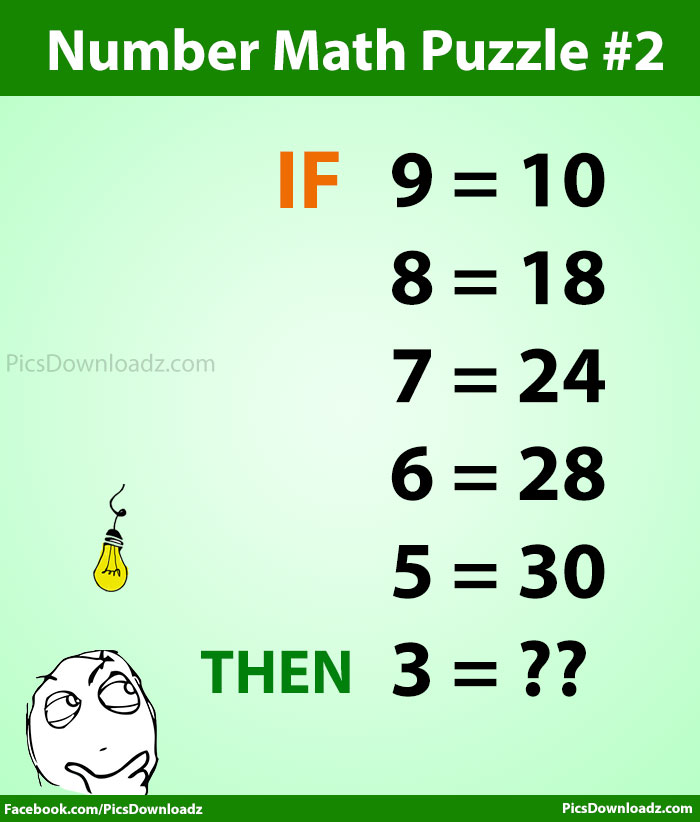This post may contain affiliate links. This means if you click on the link and purchase the item, We will receive an affiliate commission at no extra cost to you. See Our Affiliate Policy for more info.

# Can you solve this High IQ Number Math Puzzle #1, #2, #3 ? With answer

9
37559

### Solve this number math puzzle. Only 5% students solved these number puzzles correctly. Only for genius math puzzle with the answer.

Most of the people will not be able to solve these puzzles correctly. Can you solve these High IQ Intelligence number math puzzle test? Let’s find out.

Check out the equation, and find out the trick behind these number puzzles.

### Number Math Puzzle #1 (Easy)

• IF 1=3
• 2=6
• 3=9
• 4=12
• Then 5=??### Number Math Puzzle #2 (Hard)

• IF 9=10
• 8=18
• 7=24
• 6=28
• 5=30
• Then 3=??### Number Math Puzzle #3 (Very Hard)

• IF 9+1=91
• 8+2=75
• 7+3=61
• 6+4=49
• 5+5=39
• Then 3+7=??It may seem difficult at first but once you find out the trick of these math puzzles, you’ll see just how simple this math equation is.

Were you able to guess it? If so, congratulations, but don’t forget to share your answer and solution with other users in the comment section below. Don’t get it yet? don’t worry, at least you tried!

Feeling stumped and want to know the answer? Scroll down further and Please share this tricky math equation puzzle with your friends and family. Let see if they can crack this genius number puzzle question.

Number Math Puzzle #1: Answer:- 15

Number Math Puzzle #2: Answer:- 28

Number Math Puzzle #3: Answer:- 25

Search Items:-

Only For Genius Math Puzzle Problems, Math Puzzles Problems, Math Puzzles Questions, Genius Puzzles Pics, Brain Games, High IQ Picture Puzzles, Tricky Pattern Math Pics, Excellent Math Riddles With Answers and Solution, Tricky Math Riddles, Math Puzzles Problems with Answers Solutions, Riddles, Interesting Math Puzzles Problems, Math Problems Pics Solutions, Genius Math Problems Images & Answers, Only For Genius Math Riddle Pictures, Simple math puzzles, number tricks pics, Number Game images.

1.Ganesh

Number Math Puzzle #2 (Hard)

(9 x 1) + 1 = 10
(8 x 2) + 2 = 18
(7 x 3) + 3 = 24
(6 x 4) + 4 = 28
(5 x 5) + 5 = 30
(4 x 6) + 6 = 30
(3 x 7) + 7 = 28
(2 x 8) + 8 = 24
(1 x 9) + 9 = 18
(0 x 10) +10 = 10

2.Gurwinder singh

Sir how to solve #3

3.George

Number Math Puzzle #3 (Very Hard)

8+2=75

7+3=61

6+4=49

5+5=39

4+6=31

Then 3+7=25

4.Aritra Das

Number Math Puzzle#3
91-(8+2)-(8-2)= 75
75-(7+3)-(7-3)=61
61-(6+4)-(6-3)=49
49-(5+5)-(5-5)=39
39-(7+3)-|(3-7)|=25

5.Rajesh

Number math puzzle#3 solutions

8*8 +10 +1=75
7*7 +10+2=51
6*6+10+3=49
5*5+10+4=39
4*4+10+5=31
3*3+10+6=25

6.mana

9*9+1*2+8=91 8*8+2*2+7=75 7*7+3*2+6=61 6*6+4*2+5=49 5*5+5*2+5=39

•Bassem Fanari

Number Math Puzzle #3 (Very Hard)
a + b = a(a+1) + b + (b-1)
9 + 1 = (9)(10) + 1 + 0 = 91
8 + 2 = (8)(9) + 2 + 1 = 75
7 + 3 = (7)(8) + 3 + 2 = 61
6 + 4 = (6)(7) + 4 + 3 = 49
5 + 5 = (5)(6) + 5 + 4 = 39
4 + 6 = (4)(5) + 6 + 5 = 31
3 + 7 = (3)(4) + 7 + 6 = 25 (Answer)

7.Bassem Fanari

Number Math Puzzle #2 (Hard)
a = (a+1)(10-a)
9 = (10)(1) = 10
8 = (9)(2) = 18
7 = (8)(3) = 24
6 = (7)(4) = 28
5 = (6)(5) = 30
4 = (5)(6) = 30
3 = (4)(7) = 28

8.Bassem Fanari

Number Math Puzzle #1 (Easy)
a = 3a
1 = (3)(1) = 3
2 = (3)(2) = 6
3 = (3)(3) = 9
4 = (3)(4) = 12
5 = (3)(5) = 15 (Answer)